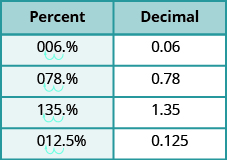Writing Decimals and Fractions as Percents

Learning Outcomes

• Convert a decimal to a percent
• Convert a fraction to a percent

To convert a decimal to a percent, remember that percent means per hundred. If we change the decimal to a fraction whose denominator is $100$, it is easy to change that fraction to a percent.

Convert a decimal to a percent.

1. Write the decimal as a fraction.
2. If the denominator of the fraction is not $100$, rewrite it as an equivalent fraction with denominator $100$.
3. Write this ratio as a percent.

example

Convert each decimal to a percent:

1. $0.05$

2.$0.83$

Solution

 1. $0.05$ Write as a fraction. The denominator is $100$. ${\Large\frac{5}{100}}$ Write this ratio as a percent. $5$%
 2. $0.83$ The denominator is $100$. ${\Large\frac{83}{100}}$ Write this ratio as a percent. $83$%

try it

To convert a mixed number to a percent, we first write it as an improper fraction.

example

Convert each decimal to a percent:
1. $1.05$

2. $0.075$

try it

Let’s summarize the results from the previous examples in the table below so we can look for a pattern.

Decimal Percent
$0.05$ $\text{5%}$
$0.83$ $\text{83%}$
$1.05$ $\text{105%}$
$0.075$ $\text{7.5%}$

Do you see the pattern? To convert a decimal to a percent, we move the decimal point two places to the right and then add the percent sign.

The next table uses the decimal numbers in the table above and shows visually to convert them to percents by moving the decimal point two places to the right and then writing the $%$ sign.In an earlier lesson, we learned how to convert fractions to decimals. Now we also know how to change decimals to percents. So to convert a fraction to a percent, we first change it to a decimal and then convert that decimal to a percent.

Convert a fraction to a percent

1. Convert the fraction to a decimal.
2. Convert the decimal to a percent.

example

Convert each fraction or mixed number to a percent:

1.  ${\Large\frac{3}{4}}$

2.  ${\Large\frac{11}{8}}$

3.  $2{\Large\frac{1}{5}}$

Convert ${\Large\frac{5}{7}}$ to a percent.

Notice that we needed to add zeros at the end of the number when moving the decimal two places to the right.

Sometimes when changing a fraction to a decimal, the division continues for many decimal places and we will round off the quotient. The number of decimal places we round to will depend on the situation. If the decimal involves money, we round to the hundredths place. For most other cases in this book we will round the number to the nearest thousandth, so the percent will be rounded to the nearest tenth.

try it

To summarize, here are the results from the previous example in a table.

Fraction Decimal Percent
${\Large\frac{3}{4}}$ $0.75$ $75$%
${\Large\frac{11}{8}}$  $1.375$ $137.5$%
$2{\Large\frac{1}{5}} ={\Large\frac{11}{5}}$  $2.20$ $220$%